# instfreq

Estimate instantaneous frequency

## Syntax

``ifq = instfreq(x,fs)``
``ifq = instfreq(x,t)``
``ifq = instfreq(xt)``
``ifq = instfreq(tfd,fd,td)``
``ifq = instfreq(___,Name,Value)``
``[ifq,t] = instfreq(___)``
``instfreq(___)``

## Description

example

````ifq = instfreq(x,fs)` estimates the instantaneous frequency of a signal, `x`, sampled at a rate `fs`. If `x` is a matrix, then the function estimates the instantaneous frequency independently for each column and returns the result in the corresponding column of `ifq`.```

example

````ifq = instfreq(x,t)` estimates the instantaneous frequency of `x` sampled at the time values stored in `t`.```

example

````ifq = instfreq(xt)` estimates the instantaneous frequency of a signal stored in the MATLAB® timetable `xt`. The function treats all variables in the timetable and all columns inside each variable independently.```

example

````ifq = instfreq(tfd,fd,td)` estimates the instantaneous frequency of the signal whose time-frequency distribution, `tfd`, is sampled at the frequency values stored in `fd` and the time values stored in `td`.```

example

````ifq = instfreq(___,Name,Value)` specifies additional options for any of the previous syntaxes using name-value pair arguments. You can specify the algorithm used to estimate the instantaneous frequency or the frequency limits used in the computation.```

example

````[ifq,t] = instfreq(___)` also returns `t`, a vector of sample times corresponding to `ifq`.```

example

````instfreq(___)` with no output arguments plots the estimated instantaneous frequency.```

## Examples

collapse all

Generate a signal sampled at 5 kHz for 4 seconds. The signal consists of a set of pulses of decreasing duration separated by regions of oscillating amplitude and fluctuating frequency with an increasing trend. Plot the signal.

```fs = 5000; t = 0:1/fs:4-1/fs; s = besselj(0,1000*(sin(2*pi*t.^2/8).^4)); plot(t,s)```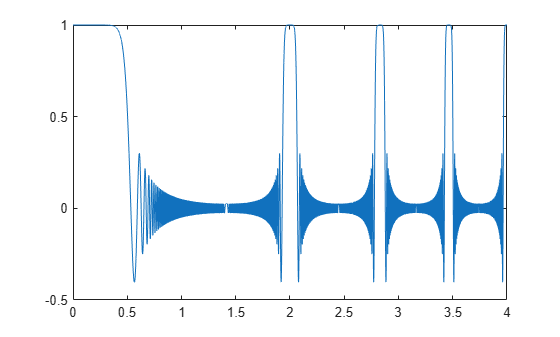Estimate the time-dependent frequency of the signal as the first moment of the power spectrogram. Plot the power spectrogram and overlay the instantaneous frequency.

`instfreq(s,fs)`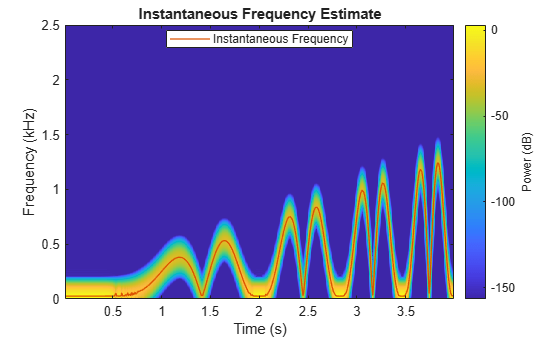Generate a complex-valued signal that consists of a chirp with sinusoidally varying frequency content. The signal is sampled at 3 kHz for 1 second and is embedded in white Gaussian noise.

```fs = 3000; t = 0:1/fs:1-1/fs; x = exp(2j*pi*100*cos(2*pi*2*t))+randn(size(t))/100;```

Estimate the time-dependent frequency of the signal as the first moment of the power spectrogram. This is the only method that `instfreq` supports for complex-valued signals. Plot the power spectrogram and overlay the instantaneous frequency.

`instfreq(x,t)`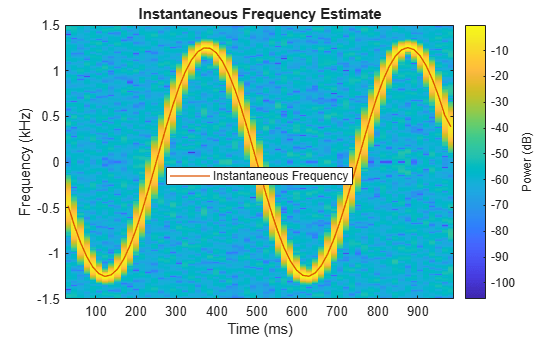Create a two-channel signal, sampled at 1 kHz for 2 seconds, consisting of two voltage-controlled oscillators. In one channel, the instantaneous frequency varies with time as a sawtooth wave whose maximum is at 75% of the period. In the other channel, the instantaneous frequency varies with time as a square wave with a duty cycle of 30%. Plot the spectrograms of the two channels. Specify a time resolution of 0.1 second for the sawtooth channel and a frequency resolution of 10 Hz for the square channel.

```fs = 1000; t = (0:1/fs:2)'; x = vco(sawtooth(2*pi*t,0.75),[0.1 0.4]*fs,fs); y = vco(square(2*pi*t,30),[0.1 0.3]*fs,fs); subplot(1,2,1) pspectrum(x,fs,'spectrogram','TimeResolution',0.1) subplot(1,2,2) pspectrum(y,fs,'spectrogram','FrequencyResolution',10)```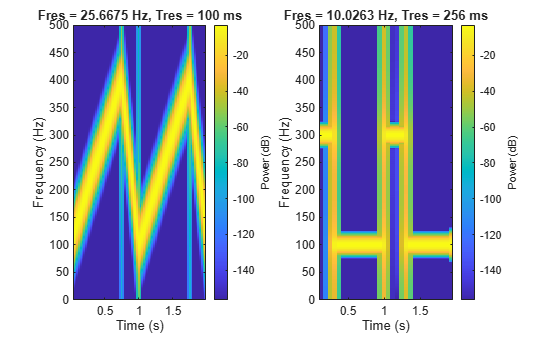Store the signal in a timetable. Compute and display the instantaneous frequency.

```xt = timetable(seconds(t),x,y); clf instfreq(xt)```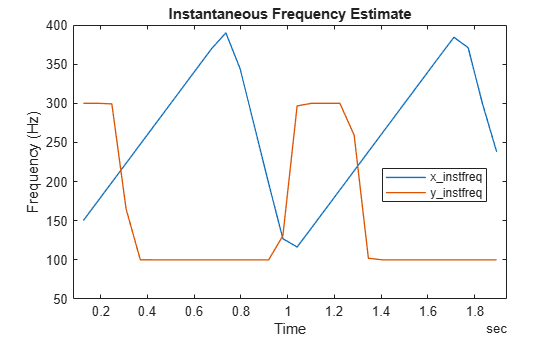Repeat the computation using the analytic signal.

`instfreq(xt,'Method','hilbert')`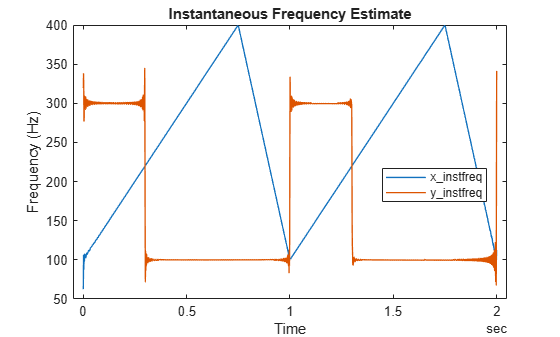Generate a quadratic chirp modulated by a Gaussian. Specify a sample rate of 2 kHz and a signal duration of 4 seconds.

```fs = 2000; t = 0:1/fs:4-1/fs; q = chirp(t-1,0,1/2,20,'quadratic',100,'convex').*exp(-1.7*(t-2).^2); plot(t,q)```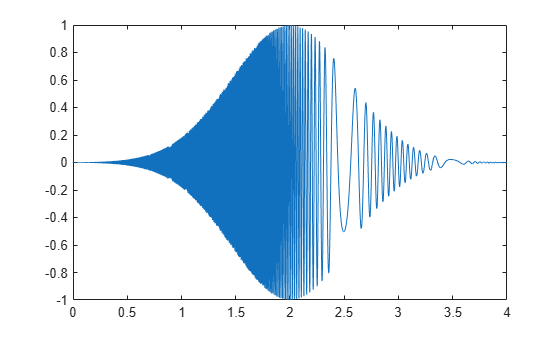Use the `pspectrum` function with default settings to estimate the power spectrum of the signal. Use the estimate to compute the instantaneous frequency.

```[p,f,t] = pspectrum(q,fs,'spectrogram'); instfreq(p,f,t)```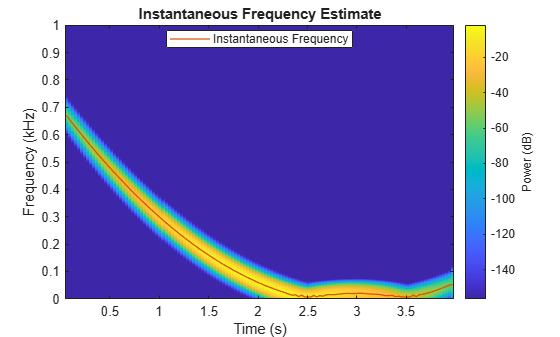Repeat the calculation using the synchrosqueezed Fourier transform. Use a 500-sample Hann window to divide the signal into segments and window them.

```[s,sf,st] = fsst(q,fs,hann(500)); instfreq(abs(s).^2,sf,st)```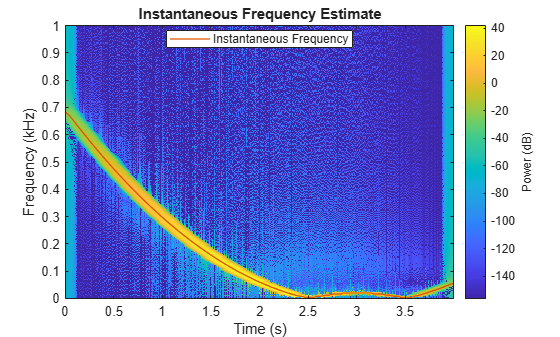Compare the instantaneous frequencies found using the two different methods.

```[psf,pst] = instfreq(p,f,t); [fsf,fst] = instfreq(abs(s).^2,sf,st); plot(fst,fsf,pst,psf)```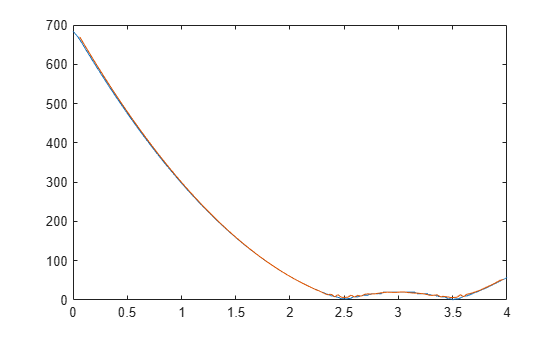Generate a sinusoidal signal sampled at 1 kHz for 0.3 second and embedded in white Gaussian noise of variance 1/16. Specify a sinusoid frequency of 200 Hz. Estimate and display the instantaneous frequency of the signal.

```fs = 1000; t = (0:1/fs:0.3-1/fs)'; x = sin(2*pi*200*t) + randn(size(t))/4; instfreq(x,t)```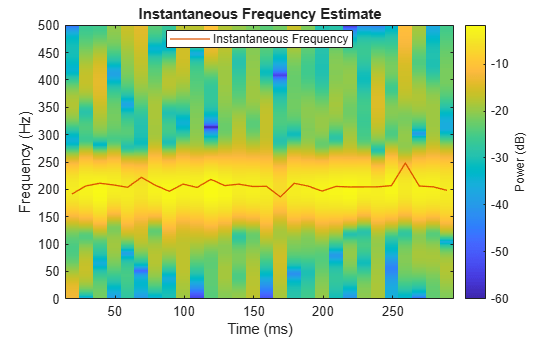Estimate the instantaneous frequency of the signal again, but now use a time-frequency distribution with a coarse frequency resolution of 25 Hz as input.

```[p,fd,td] = pspectrum(x,t,'spectrogram','FrequencyResolution',25); instfreq(p,fd,td)```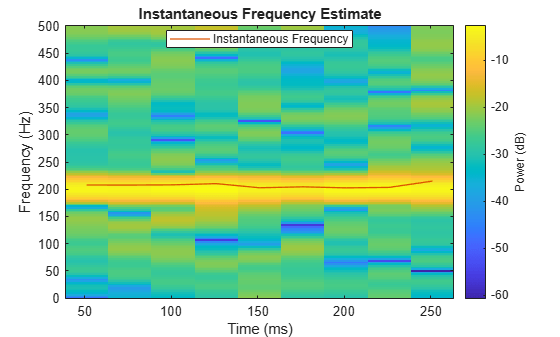## Input Arguments

collapse all

Input signal, specified as a vector or matrix. If `x` is a vector, then `instfreq` treats it as a single channel. If `x` is a matrix, then `instfreq` computes the instantaneous frequency independently for each column and returns the result in the corresponding column of `ifq`.

Example: `sin(2*pi*(0:127)/16)+randn(1,128)/100` specifies a noisy sinusoid

Example: `[2 1].*sin(2*pi*(0:127)'./[16 64])` specifies a two-channel sinusoid.

Data Types: `single` | `double`
Complex Number Support: Yes

Sample rate, specified as a positive scalar. The sample rate is the number of samples per unit time. If the unit of time is seconds, then the sample rate is in Hz.

Data Types: `single` | `double`

Sample times, specified as a real vector, a `duration` scalar, a `duration` array, or a `datetime` array.

• `duration` scalar — The time interval between consecutive samples of `x`.

• Real vector, `duration` array, or `datetime` array — The time instant corresponding to each element of `x`.

Example: `seconds(1)` specifies a 1-second lapse between consecutive measurements of a signal.

Example: `seconds(0:8)` specifies that a signal is sampled at 1 Hz for 8 seconds.

Data Types: `single` | `double` | `duration` | `datetime`

Input timetable. `xt` must contain increasing, finite row times.

If a timetable has missing or duplicate time points, you can fix it using the tips in Clean Timetable with Missing, Duplicate, or Nonuniform Times.

Example: `timetable(seconds(0:4)',randn(5,1))` specifies a random process sampled at 1 Hz for 4 seconds.

Example: `timetable(seconds(0:4)',randn(5,3),randn(5,4))` contains a three-channel random process and a four-channel random process, both sampled at 1 Hz for 4 seconds.

Data Types: `single` | `double`
Complex Number Support: Yes

Time-frequency distribution, specified as a matrix sampled at the frequencies stored in `fd` and the time values stored in `td`. This input argument is supported only when `'Method'` is set to `'tfmoment'`.

Example: ```[p,f,t] = pspectrum(sin(2*pi*(0:511)/4),4,'spectrogram')``` specifies the time-frequency distribution of a 1 Hz sinusoid sampled at 4 Hz for 128 seconds, and also the frequencies and times at which it is computed.

Data Types: `single` | `double`

Frequency and time values for time-frequency distribution, specified as vectors. These input arguments are supported only when `'Method'` is set to `'tfmoment'`.

Example: ```[p,f,t] = pspectrum(sin(2*pi*(0:511)/4),4,'spectrogram')``` specifies the time-frequency distribution of a 1 Hz sinusoid sampled at 4 Hz for 128 seconds, and also the frequencies and times at which it is computed.

Data Types: `single` | `double`

### Name-Value Pair Arguments

Specify optional comma-separated pairs of `Name,Value` arguments. `Name` is the argument name and `Value` is the corresponding value. `Name` must appear inside quotes. You can specify several name and value pair arguments in any order as `Name1,Value1,...,NameN,ValueN`.

Example: `'Method','tfmoment','FrequencyLimits',[25 50]` computes the instantaneous frequency of the input in the range from 25 Hz to 50 Hz by finding the first conditional spectral moment of the time-frequency distribution.

Frequency range, specified as the comma-separated pair consisting of `'FrequencyLimits'` and a two-element vector in Hz. If not specified, `'FrequencyLimits'` defaults to `[0 fs/2]` for real-valued signals and to `[-fs/2 fs/2]` for complex-valued signals. This argument is supported only when `'Method'` is set to `'tfmoment'`.

Data Types: `single` | `double`

Computation method, specified as the comma-separated pair consisting of `'Method'` and either `'tfmoment'` or `'hilbert'`.

• `'tfmoment'` — Compute the instantaneous frequency as the first conditional spectral moment of the time-frequency distribution of `x`. If `x` is nonuniformly sampled, then `instfreq` interpolates the signal to a uniform grid to compute instantaneous frequencies.

• `'hilbert'` — Compute the instantaneous frequency as the derivative of the phase of the analytic signal of `x` found using the Hilbert transform. This method accepts only uniformly sampled, real-valued signals and does not support time-frequency distribution input.

## Output Arguments

collapse all

Instantaneous frequency, returned as a vector, a matrix, or a timetable with the same dimensions as the input.

Times of frequency estimates, returned as a real vector, a `duration` array, or a `datetime` array.

collapse all

### Instantaneous Frequency

The instantaneous frequency of a nonstationary signal is a time-varying parameter that relates to the average of the frequencies present in the signal as it evolves , .

• If `'Method'` is set to `'tfmoment'`, then `instfreq` estimates the instantaneous frequency as the first conditional spectral moment of the time-frequency distribution of the input signal. The function:

1. Computes the spectrogram power spectrum P(t,f), of the input using the `pspectrum` function and uses the spectrum as a time-frequency distribution.

2. Estimates the instantaneous frequency using

`${f}_{\text{inst}}\left(t\right)=\frac{{\int }_{0}^{\infty }f\text{\hspace{0.17em}}P\left(t,f\right)\text{\hspace{0.17em}}df}{{\int }_{0}^{\infty }P\left(t,f\right)\text{\hspace{0.17em}}df}.$`

• If `'Method'` is set to `'hilbert'`, then `instfreq` estimates the instantaneous frequency as the derivative of the phase of the analytic signal of the input. The function:

1. Computes the analytic signal, xA, of the input using the `hilbert` function.

2. Estimates the instantaneous frequency using

`${f}_{\text{inst}}\left(t\right)=\frac{1}{2\pi }\frac{d\varphi }{dt},$`

where ϕ is the phase of the analytic signal of the input.

 Boashash, Boualem. "Estimating and Interpreting The Instantaneous Frequency of a Signal—Part 1: Fundamentals." Proceedings of the IEEE®. Vol. 80, April 1992, pp. 520–538.

 Boashash, Boualem. "Estimating and Interpreting The Instantaneous Frequency of a Signal—Part 2: Algorithms and Applications." Proceedings of the IEEE. Vol. 80, April 1992, pp. 540–568.